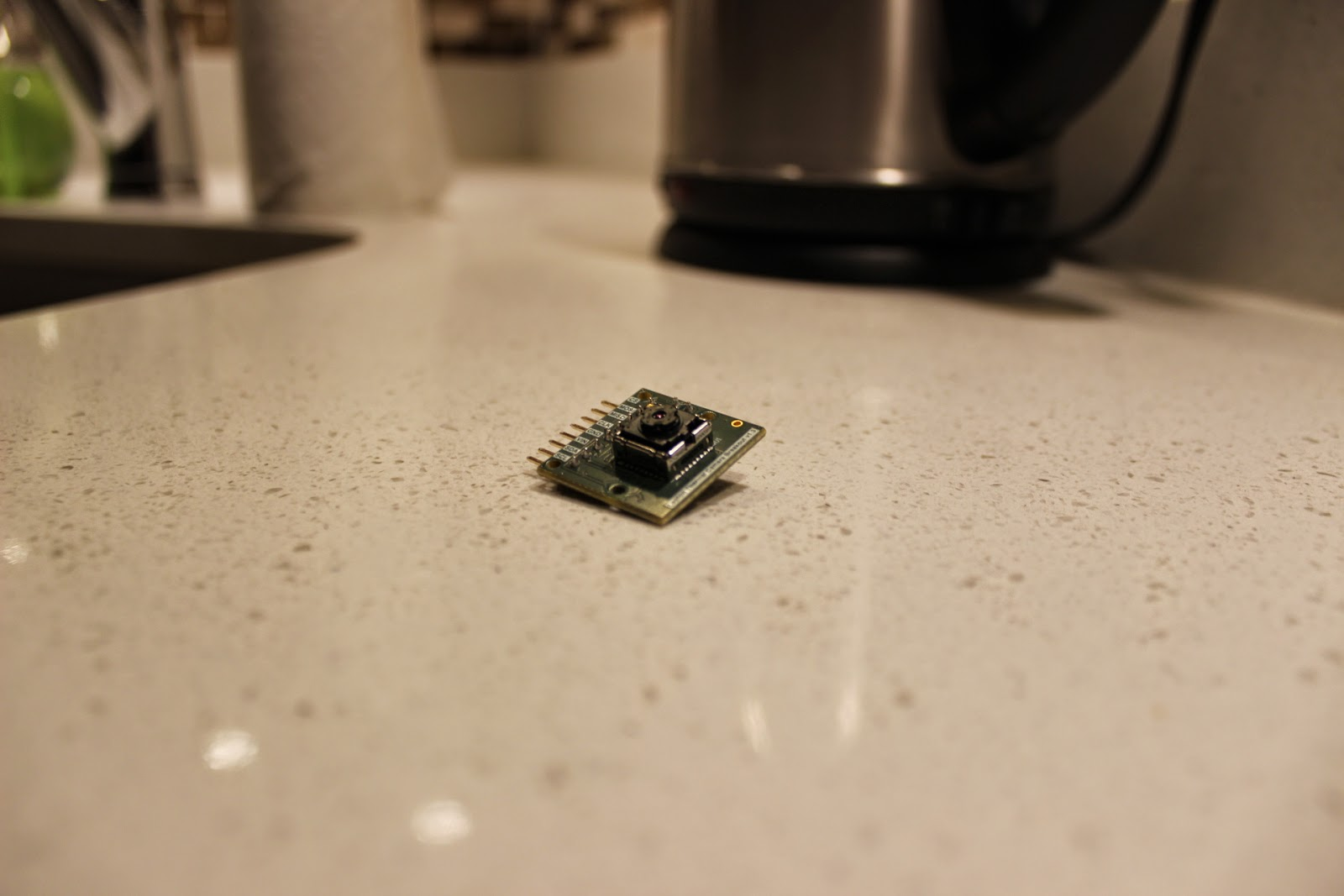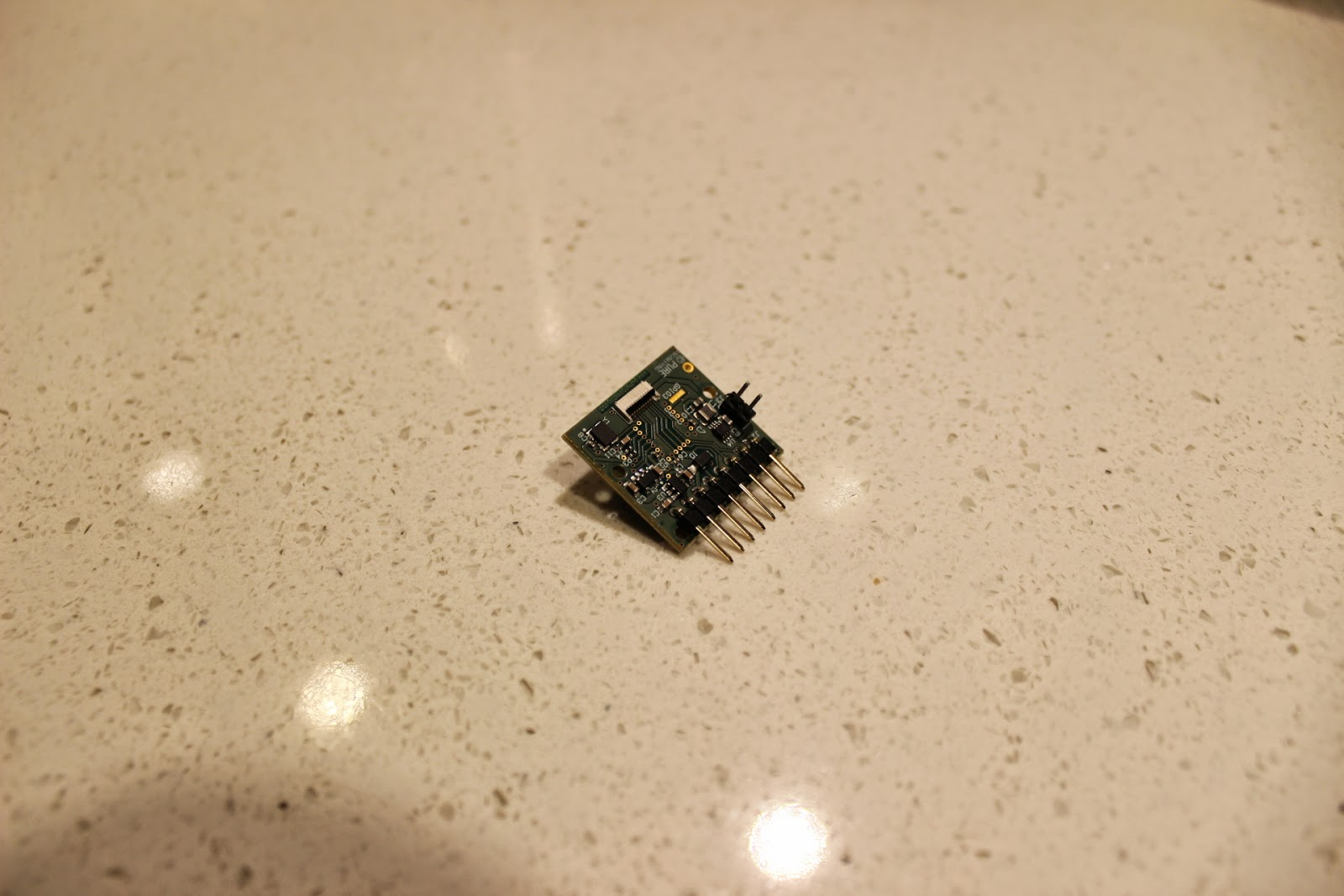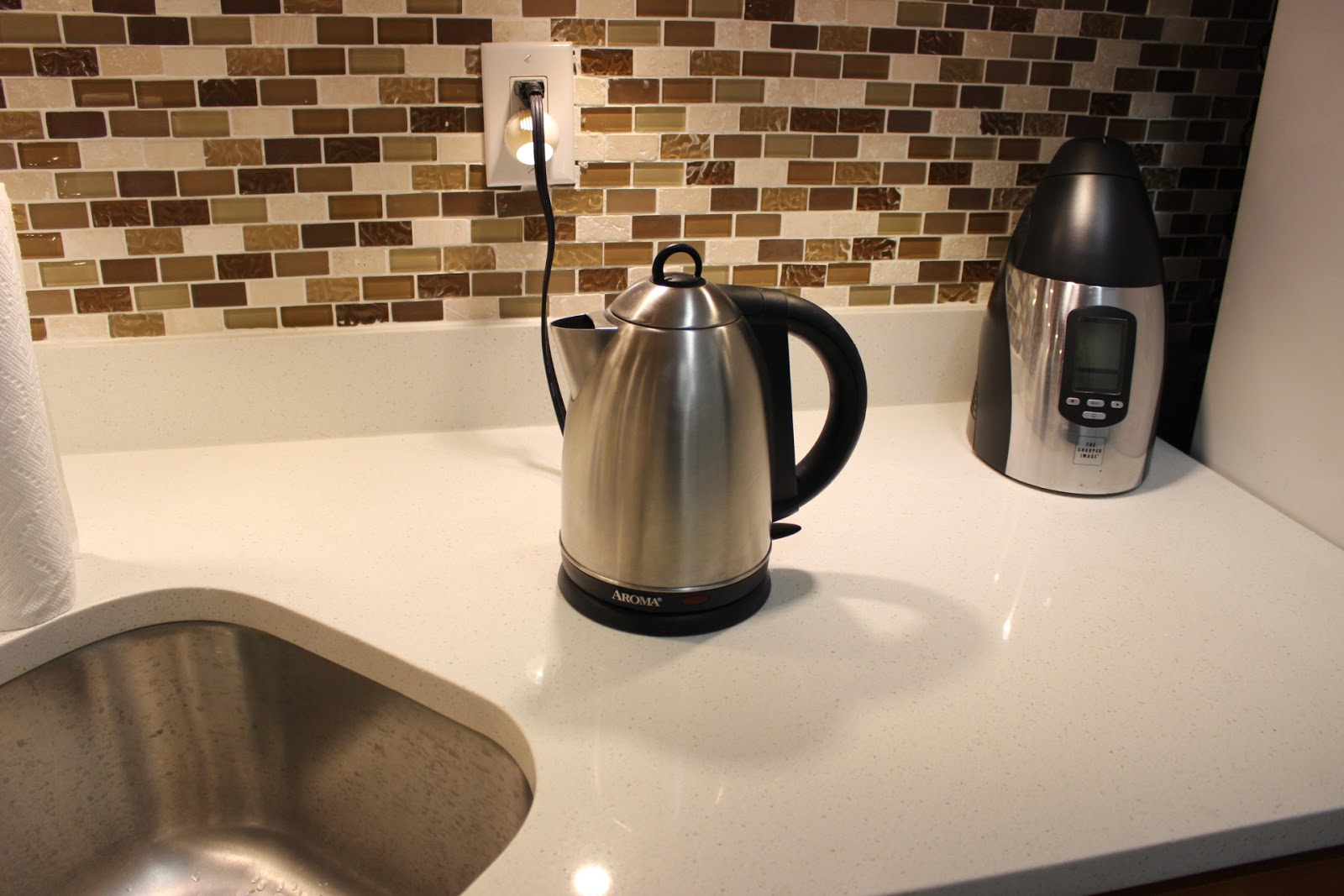## Sunday, November 23, 2014

### Flir Lepton Thermal Imaging Sensor + Gameduino 2

I recently got my hands on a pair of Flir Lepton thermal imaging sensors and have spent the last week bringing them online in my spare time. These are absolutely incredible devices that I believe will pave the way to consumer devices incorporating thermal imaging cameras. The footprint of the camera module (and optical assembly) is about the size of a dime. The resolution is 80x60 at 14bpp which is remarkable despite sounding low.Thermal Andrew :]
I have successfully implemented a driver for the Lepton module and displayed frames on an LCD. This is all running on an STM32F4 processor on a Nucleo board. Attached to it is a Gameduino 2 which incorporates the FT800 graphics processor. I have implemented my own colorization and min/max scaling before uploading the frames to the GPU.Front System Overview
I have used some simple jumper wires to interface with this camera. This setup is running at 21MHz with no issues. I am using a breakout board provided by Pure Engineering. You can pick up one from Tindie if you are interested. The Lepton module can be ripped out the Flir One iPhone accessory for now.Rear System Overview and Second Camera (future project ;])
I wrote my own driver for the Lepton core and the FT800 graphics processor. Continue reading for more details!

### Hardware Overview

As I mentioned, I am using the Flir Lepton sensor and a breakout board designed by Pure Engineering.Lepton seated in the Pure Engineering breakout boardBreakout Board Rear

### Protocol and Driver Implementation

This module incorporates two interfaces. One is i2c and the other is SPI. The i2c interface is used to configure the module in a number of ways. This is something that I have not explored yet. In the default configuration of this camera, it will export frame data over SPI. Each packet is 164 bytes long. The first two bytes are line number, the second two bytes are a CRC and the final 160 bytes are the pixel data for that line. Each pixel is sent as two bytes to accommodate the 14-bit range. Data is sent in big-endian format (high byte first).VoSPI Packet
Occasionally the camera will send a synchronization packet. In this case, line number is sent as xFxx. The x bits are "don't cares" so this can be tested for by applying a bitmask to the first byte of 0x0F and testing that it is equal to 0x0F.

I defined some symbols to make parsing the protocol easier.
#define LEPTON_PACKET_ID_LENGTH 2
#define LEPTON_PACKET_CRC_LENGTH 2
#define LEPTON_PACKET_CONTENT_LENGTH 160

(LEPTON_PACKET_ID_LENGTH + LEPTON_PACKET_CRC_LENGTH)
#define LEPTON_PACKET_LENGTH \

#define LEPTON_WIDTH 80
#define LEPTON_HEIGHT 60

I defined a Lepton_t struct to maintain a reference to its' SPI module and chip select line. I also have a LeptonColor_t struct to store a simple RGB565 color that the FT800 can use.
typedef struct Lepton_t {
volatile SystemSpiModule_t *Spi;
volatile SystemGpioModule_t *CsPort;
volatile uint32_t CsPin;
uint8_t FrameBuffer[LEPTON_HEIGHT * LEPTON_PACKET_CONTENT_LENGTH];
} Lepton_t;

typedef struct LeptonColor_t {
struct {
uint8_t red : 5;
uint8_t green : 6;
uint8_t blue : 5;
};
} LeptonColor_t;

The API for this driver is very simple.
/*
* Initializes the Lepton module
*/
void LeptonInit(Lepton_t *lepton);

/*
* Reads a frame into the Lepton_t framebuffer.
*/

/*
* Returns a pixel from the frame buffer at a given x, y
*/
uint16_t LeptonReadPixel(Lepton_t *lepton, uint8_t x, uint8_t y);

Allocating a FrameBuffer as part of the Lepton_t struct was a decision I made to keep the driver self-contained. It is certainly possible to refactor this code and not buffer the entire frame.

The implementation of this driver is simple. There are a few private utility functions to start/end transfers, reset the camera and read various amounts of data.
/* Lepton Private Functions ***************************************************/

inline void LeptonBeginTransfer(Lepton_t *lepton) {
lepton->CsPort->Output.Port &= ~(1 << lepton->CsPin);
}

inline void LeptonEndTransfer(Lepton_t *lepton) {
lepton->CsPort->Output.Port |= (1 << lepton->CsPin);
}

inline void LeptonReset(Lepton_t *lepton) {
LeptonEndTransfer(lepton);
for(volatile int i = 0; i < 100000; i++);
}

lepton->Spi->Data = 0x00;
while(!lepton->Spi->Status.RxFull);
return lepton->Spi->Data;
}

inline bool LeptonReadLine(Lepton_t *lepton, uint8_t line, uint8_t *buffer) {
bool success = true;
LeptonBeginTransfer(lepton);

for(int i = 0; i < LEPTON_PACKET_HEADER_LENGTH; i++) {
}

if((buffer & 0x0F) == 0x0F) {
success = false;
} else if(buffer != line) {
success = false;
}

for(int i = 0; i < LEPTON_PACKET_CONTENT_LENGTH; i++) {
}

LeptonEndTransfer(lepton);
return success;
}
These utility functions are assembled to create the Lepton driver that is exposed in Lepton.h.
/* Lepton.h Implementations ***************************************************/

void LeptonInit(Lepton_t *lepton) {
// Setup the SPI Module
lepton->Spi->Config.SlaveManageEnable = true;
lepton->Spi->Config.InternalSelect = true;
lepton->Spi->Config.DeviceMode = SystemSpiDeviceMode_Master;
lepton->Spi->Config.Prescaler = SystemSpiPrescaler_2;
lepton->Spi->Config.ClockPhase = SystemSpiClockPhase_Second;
lepton->Spi->Config.ClockIdle = SystemSpiClockIdle_High;
lepton->Spi->Config.Enabled = true;

LeptonReset(lepton);
}

for(int i = 0; i < LEPTON_HEIGHT; i++) {
&lepton->FrameBuffer[i * LEPTON_PACKET_CONTENT_LENGTH])) {
i--;
}
}
}

uint16_t LeptonReadPixel(Lepton_t *lepton, uint8_t x, uint8_t y) {
uint16_t pixelIndex = (y * LEPTON_PACKET_CONTENT_LENGTH) + (2 * x);

uint8_t high_byte = lepton->FrameBuffer[pixelIndex];
uint8_t low_byte = lepton->FrameBuffer[pixelIndex + 1];

return (high_byte << 8) | low_byte;
}
This camera uses SPI mode 3 which means that you must sample on the second edge of an idle-high clock. I am clocking my camera at 21MHz which is just a slight overclock.

### Generating Pseudocolor Images

In the image below you can see a few fascinating details about boiling water in a kettle. You can see the hot steam that is flowing out of the spout. You can also see that the power cord is quite warm (relatively speaking).Boiling KettleKettle
As you can see, the pseudocolor image exposes some interesting information about the scene. Generating a pseudocolor involves selecting colors from a gradient. For example, you could map black to the coolest object in your scene, red to the hottest and ignore the green and blue channels.Red Black Gradient

There are basically two ways to display thermal image data. The first is as a grayscale image. This has the benefit of introducing no ambiguity into the image that we view. This is quite common in military applications. The second way is to apply a colorization algorithm to generate a pseudocolor image. This uses the bits in our display more cleverly to display a wider range of temperatures.

Consider a grayscale image on an 24-bit RGB display. In this context you would only be able to see 256 levels of temperature. If you apply false-colorization and display the image on a 24-bit RGB display you have the potential to display a much greater number of levels of temperature. As an example, I am using a rainbow to colorize my image which provides 1792 levels.
Black - Red    : 256 Levels - Coldest
Red - Yellow   : 256 Levels
Yellow - Green : 256 Levels
Green - Cyan   : 256 Levels
Cyan - Blue    : 256 Levels
Blue - Violet  : 256 Levels
Violet - White : 256 Levels - Hottest

You can see that the advantage to using a pseudocolor image is very clear for most applications.Rainbow Gradient
To generate a colorized image, I first find the minimum and maximum values for a given image.
LeptonReadFrame(&camera);

// Compute the min/max values of this frame.
int min = 16384;
int max = 0;

for(int i = 0; i < LEPTON_HEIGHT - 1; i++) {
for(int j = 0; j < LEPTON_WIDTH; j++) {
uint16_t value = LeptonReadPixel(&camera, j, i);

if(value < min) {
min = value;
}

if(value > max) {
max = value;
}
}
}
I generated an RGB565 lookup table of colors in C#. This table has 320 entries and is loaded into flash memory.
const LeptonColor_t colors[] = {
{ .red = 0, .green = 0, .blue = 0 },
{ .red = 1, .green = 0, .blue = 0 },
{ .red = 2, .green = 0, .blue = 0 },
{ .red = 3, .green = 0, .blue = 0 },
{ .red = 4, .green = 0, .blue = 0 },
{ .red = 5, .green = 0, .blue = 0 },
{ .red = 6, .green = 0, .blue = 0 },
{ .red = 7, .green = 0, .blue = 0 },
{ .red = 8, .green = 0, .blue = 0 },
{ .red = 9, .green = 0, .blue = 0 },

// ...

{ .red = 31, .green = 50, .blue = 31 },
{ .red = 31, .green = 51, .blue = 31 },
{ .red = 31, .green = 52, .blue = 31 },
{ .red = 31, .green = 53, .blue = 31 },
{ .red = 31, .green = 54, .blue = 31 },
{ .red = 31, .green = 55, .blue = 31 },
{ .red = 31, .green = 56, .blue = 31 },
{ .red = 31, .green = 57, .blue = 31 },
{ .red = 31, .green = 58, .blue = 31 },
{ .red = 31, .green = 59, .blue = 31 },
{ .red = 31, .green = 60, .blue = 31 },
{ .red = 31, .green = 61, .blue = 31 },
{ .red = 31, .green = 62, .blue = 31 },
{ .red = 31, .green = 63, .blue = 31 },
};
This allows me to lookup the corresponding color for a given normalized temperature value very quickly. I scale the value of each pixel to be within range of the color lookup table.
// Upload the frame to the GPU.
for(int i = 0; i < LEPTON_HEIGHT; i++) {
for(int j = 0; j < LEPTON_WIDTH; j++) {
uint16_t value = LeptonReadPixel(&camera, j, i);

int scaled_value = (320 * (value - min)) / (max - min);
scaled_value = scaled_value < 0 ? 0 : scaled_value;
scaled_value = scaled_value > 319 ? 319 : scaled_value;

LeptonColor_t color = colors[scaled_value];

// ...

Using this technique I can utilize my LCD to display a wider range of temperature values.A "hot" apartment building against the cold NYC sky :]

### Caveats of Interpolating Pseudocolor Images

If you are planning to use interpolation to scale up the images captured by the Lepton sensor, pay careful attention to how you interpolate the data.

#### Nearest Neighbour Interpolation

If you take the naive approach and use nearest neighbour to scale up the images, you do not need to be concerned. The result will accurately reflect the captured images in pseudocolor but will be larger in size.

#### Bilinear/Linear/Sinc Interpolation

If you decide to use a better interpolation algorithm like bilinear, linear or sinc you must generate the final image by interpolating from the 80x60x14bpp sensor data directly. If you generate a pseudocolor image at 80x60 and scale it up, the result will not be accurate.

Imagine that you have two objects next to one another. One object is red and the other is blue. The natural interpolation between these colors is to transition through violet. This is incorrect! If you interpolate the data as temperature values they should interpolate through yellow, green, cyan and finally blue. You are displaying the region between these two objects as hotter than both of them.Interpolation Error!
This has been a fun experiment and I am looking forward to working on more projects with this sensor.Flir Lepton + Gameduino + Nucleo running on a portable USB power supply :]